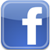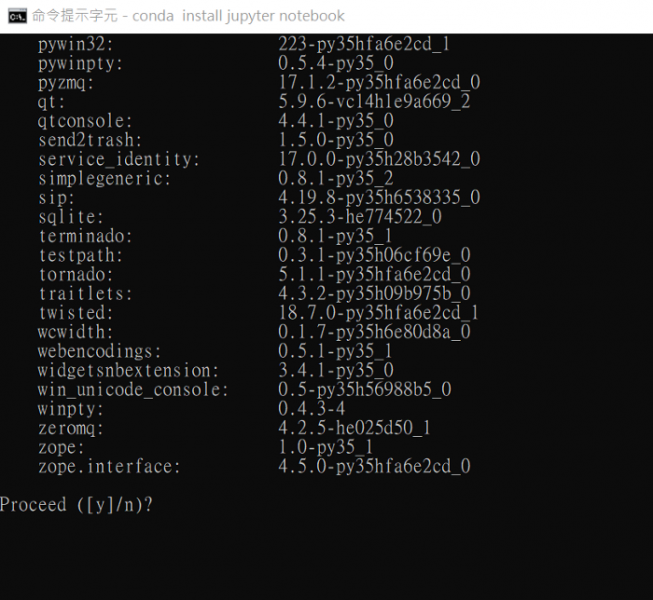##帳號 自動登錄找回密碼 密碼 註冊

# [教學] 在 Anaconda 虛擬環境下安裝 Tensorflow 與 Keras發表於 2020-9-29 11:39:22 | 顯示全部樓層 |閱讀模式Anaconda 虛擬環境 Tensorflow Keras Python深度學習 ( Deep Learning ) 是機器學習的一個分支，也是人工智慧領域中成長最快速與應用最廣泛的一個學科。如果我們想要學習深度學習模型，可以再另外安裝 Tensorflow 和 Keras 這兩套深度學習套件。為了配合 Tensorflow 所支援的 Python 版本，我們可以利用 Anaconda 虛擬環境來產生全新的 Python 環境，以避免 Anaconda 裡新版的 Python 版本可能與 Tensorflow 會的相容性問題。利用命令提示字元輸入命令視窗建立tensorflow虛擬環境。(1.) 按下搜尋圖示，輸入cmd，點選 [命令提示字元] 以啟動命令提示字元視窗。 Anaconda 虛擬環境 Tensorflow Keras Python(2) 建立工作資料夾pythonwork，並且切換進入這個工作資料夾裡：以後如果要儲存的東西，都會在同一個資料夾，在找尋資料的時候比較方便，database 也會比較好備份。md \pythonwork cd \pythonwork複製代碼 前面加斜線的意思是根目錄，就不會在現在的資料夾往下建立，而是直接在根目錄建立 pythonwork 這個資料夾。 Anaconda 虛擬環境 Tensorflow Keras Python(3.) 接下來，使用 conda 命令來建立一個命名為 tensorflow 的虛擬環境，並在裡面安裝 Python 3.5 版本。接下來再用這個虛擬環境來安裝 tensorflow 的 CPU 版本。conda create --name tensorflow python=3.5複製代碼 執行畫面如下 : Anaconda 虛擬環境 Tensorflow Keras Python建立一個命名為tensorflow的虛擬環境畫面會出現 “Proceed([y]/n)?”，按下 y 之後，會開始安裝tensorflow虛 擬環境，並且安裝套件。 Anaconda 虛擬環境 Tensorflow Keras Python執行畫面會出現Proceed([y]/n)? 按下 “y”執行安裝利用activate tensorflow 命令啟動tensorflow的anaconda虛擬環境(若要 關閉虛擬環境則是使用deactivate tensorflow): 進入前到Anaconda點選 CMD.EXE prompt Anaconda 虛擬環境 Tensorflow Keras Pythonconda activate tensorflow複製代碼 Anaconda 虛擬環境 Tensorflow Keras Python啟動tensorflow的anaconda虛擬環境安裝 Tensorflow : 選哪個都可conda install tensorflow複製代碼pip install tensorflow複製代碼 畫面一樣會出現 “Proceed([y]/n)?”，按下 y 之後，開始安裝。 Anaconda 虛擬環境 Tensorflow Keras Python安裝 Tensorflow cpu or gpu: pip install tensorflow-gpu複製代碼pip install tensorflow-cpu 複製代碼 安裝 Keras :conda install -c conda-forge keras複製代碼 畫面一樣會出現 “Proceed([y]/n)?”，按下 y 之後，開始安裝。 Anaconda 虛擬環境 Tensorflow Keras Python在 tensorflow 的虛擬環境底下安裝 jupyter notebook。conda install jupyter notebook複製代碼 Anaconda 虛擬環境 Tensorflow Keras Python按下 y 安裝 jupyter notebook(4.) 在 Jupyter Notebook 中輸入測試碼來測試 Tensorflow 是否安裝成功。在已安裝好的 Anaconda 資料夾中開啟剛剛安裝的 Jupter Notebook(tensorflow)。 Anaconda 虛擬環境 Tensorflow Keras Python在已安裝好的 Anaconda 資料夾中開啟剛剛安裝的 Jupter Notebook(tensorflow)。進入到 Jupyter Notebook 的頁面後，按下右上方的 New >> Python3，開啟直譯器的編輯畫面。 Anaconda 虛擬環境 Tensorflow Keras Python在新的 cell 中輸入以下程式碼，確認是否已經成功安裝 Tensorflow。import tensorflow as tf hello = tf.constant("Hello, TensorFlow!") sess = tf.Session() print(sess.run(hello))複製代碼 按下 shift + enter 或是上方的 Run 按鈕執行這幾行敘述。 執行結果如下，代表 Tensorflow 已經成功安裝。 Anaconda 虛擬環境 Tensorflow Keras Python這樣就安裝好 TensorFlow 、並且可以執行了 ! 文章出處 網頁設計,網站架設 ,網路行銷,網頁優化,SEO - NetYea 網頁設計

 本版積分規則 回帖後跳轉到最後一頁
﻿

GMT+8, 2020-10-23 01:17 , Processed in 0.059747 second(s), 24 queries .